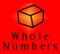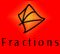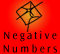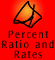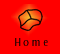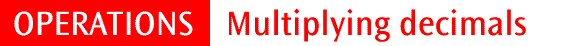| Multiplying a decimal by a whole number |
| Multiplying decimals by decimals |
| Rule for multiplying decimals | Quick Quiz |

Multiplication of decimals relies on knowing how to multiply whole numbers, understanding place value, and appreciating the various multiplication situations involving decimals. The endless base ten chain shows the consistency of the base ten place value system from whole numbers to decimals.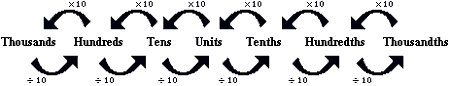As with whole number multiplication, the order of the numbers being multiplied does not affect the product. This is called the commutative property of multiplication.

Multiplying a decimal by a whole number

Multiplying decimals relies on adapting multiplication of whole numbers. When one of the numbers being multiplied is reduced to a tenth of its original size, the product is also reduced to a tenth of its original size. The following chart demonstrates this relationship.

 Example Working out Regrouping Answer 3 x 8 = 24 Three groups of eight ones is 24 ones. 24 ones is the same as 2 tens and 4 ones. 24 3 x 0.8 = 2.4 Three groups of eight tenths is 24 tenths. 24 tenths is the same as 2 ones and 4 tenths. 2.4 3 x 0.08 = 0.24 Three groups of eight hundredths is 24 hundredths. 24 hundredths is the same as 2 tenths and 4 hundredths. 0.24 3 x 0.008 = 0.024 Three groups of eight thousandths is 24 thousandths. 24 thousandths is the same as 2 hundredths and 4 thousandths. 0.024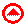Multiplying decimals by decimals

When multiplying a number by a decimal less than one, the product will be smaller than the number being multiplied. This is because we are finding a fractional amount of a quantity. For example, 0.1 x 0.8 = 0.08, because the question is asking us to find one tenth of eight tenths. A tenth of a tenth (or a tenth multiplied by a tenth) is a hundredth, thus one tenth of eight tenths is eight hundredths.

Viewing decimal multiplication problems in common fraction and extended decimal form may help us better understand the answer. We can link multiplication of decimals to common fractions because we know that 0.1 (one tenth) is the same as 1/10, or 1 ÷ 10. Similarly, we can expand the way we record decimals to gain an understanding of the size of answers because we know that 0.1 is the same as 1 x 0.1, or 1 ÷ 10. We can rewrite 0.1 x 0.8 as:

• 1/10 x 8/10 = 8/100 = 0.08 (using rules for fraction multiplication)
• 0.1 x (0.1 x 8) = 0.01 x 8 = 0.08 (using rules for decimal multiplication)
• one tenth of eight tenths is eight hundredths (using common sense)

The relationship between the place value of the numbers being multiplied and the product is summarised by the following chart. As we move to the right or down the chart, the numbers increase by a factor of ten, so the answers increase by a factor of ten.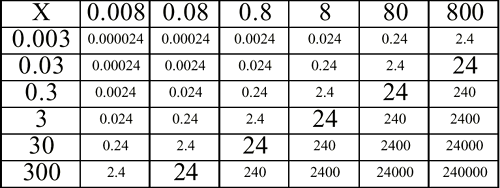Rule for multiplying decimals

A common way to multiply decimals is to treat them as whole numbers, and then position the decimal point in the product. The number of digits after the decimal points in the factors determines where the decimal point is placed in the answer.

For example, 0.3 x 0.8 = 0.24. There is one digit after the decimal point in both 0.3 and 0.8, two digits altogether, so the answer will have two digits after the decimal point. We know that 3 x 8 = 24, so we can place the decimal point in front of two digits, giving 0.24. This method relies on a sound understanding of place value to check that answers are reasonable.

 Example Correct placement of the decimal point Place value thinking 0.4 x 0.5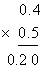This is just like 4 x 5 4 x 5 = 20 0.4 has one decimal place, 0.5 has one decimal place, so my answer will have two decimal places. My answer is 0.20 This is 4 tenths of 5 tenths. Tenths by tenths gives hundredths, so my answer will have two decimal places. 4 tenths x 5 tenths = 20 hundredths 0.4 x 0.5 = 0.20

Example 1: Full explanation of 1.8 x 2.3 = 4.14

A wire expands to 1.8 times its length when heated. The wire is 2.3 cm long. How long will it be when heated?

 Example Working out Thinking 1.8 x 2.3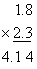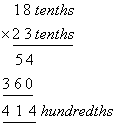1.8 x 2.3 = 4.14 The wire will be 4.14 cm long This is nearly 2 times 2.3 cm, so the answer will be about 4 cm. The easiest way to do this example is to use whole number multiplication and then adjust our answer accordingly. To be able to multiply 18 by 23 we multiply 1.8 by 10 and 2.3 by 10 and so have actually multiplied by 100. (1.8 x 10 x 2.3 x 10 = 18 x 23 x 100) Once we have done the whole number multiplication, we must then divide our answer by 100 to compensate for this. 18 x 23 = 414 Out final answer is one hundredth of 18 x 23. So 1.8 x 2.3 = 4.14 This answer makes sense according to my initial estimation .

Example 2: Placing the decimal point, 23 x 0.67 = 15.41

Petrol costs \$0.67 per litre. Khumalo pumps 23 litres of petrol into his car. How much does it cost him? To find this out I need to multiply 23 litres by \$0.67. 0.67 is over half of one dollar, so my answer will be over \$11 (half of \$22).

Example 3: Full explanation of 0.234 x 0.07 = 0.01638

 Example Working out Thinking 0.234 x 0.07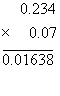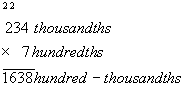We can use whole number multiplication to solve this problem if we are aware of how decimals relate to whole numbers. 0.234 can be expressed as 234 thousandths and 0.07 can be expressed as 7 hundredths. This can be confirmed using a number expander (discussed in Meaning and Models). We then do the multiplication as whole number multiplication. Because we are multiplying thousandths and hundredths, our answer will be in hundred-thousandths. We now need to express 1638 hundred-thousandths as a decimal. If we are not sure how to do this we can use the number expander.

The following movie shows a 'procedural' method being used to solve this problem.

Rows of zeros contribute nothing to the final answer and therefore are a waste of time and ink. The decimal point can be placed without multiplying the rows of zeros. 234 x 7 is 1638, but 0.237 x 0.07 will have 5 decimal places in the answer, so it will be 0.01638.

Caution: if you use the rule of counting decimal places in the question to find the number of decimal places in the answer, you MUST not discard zeros too early. For example, to multiply 0.25 by 0.4, you need 2+1 decimal places and the multiplication without a decimal point gives 0100. The answer is therefore 0.100. This is equal to 0.1, but the right-most decimal places cannot be discarded until after the multiplication rule has been used.

 Why is it not necessary to line up the decimal point to multiply? In addition and subtraction, it is important to line up the place value columns because we can only add or subtract digits in the same place value columns. We can, however, multiply digits in one place value column by those in another, so we do not need to line up the columns.Multiplication Quiz

1. Given that 4857 x 6 = 29142, find:

 (a) 4857 x 0.6 (b) 48.57 x 0.6 (c) 485.7 x 0.0006

 2 1.5 x 7.62 3 0.4 x 0.06 4 0.002 x 0.05

Extra questions:

1. Find the product of 7.15 and 1.9

2. Evaluate 7.04 x 1.1

3. Steve’s stride is 84.6 cm. How many centimetres will he travel if he takes 200 strides?

4. The length of my front garden bed is 4.3 times longer than the length of my back garden bed. If the length of my back garden bed is 156 cm, how long is my front garden bed?

5. Is the following statement true or false: 9.6734 x 0.9 > 9.6734?

If you would like to do some more questions, click here to go to the mixed operations quiz at the end of the division section.